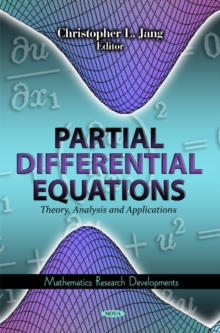Supporting your high street Find out how »
• My AccountBasket# Partial Differential Equations : Theory, Analysis & Applications Hardback

## Edited by Christopher L. Jang

#### Description

Partial differential equations are used to formulate and thus aid the solution of problems involving functions of several variables, such as the propagation of sound or heat, electrostatics, electrodynamics, fluid flow, and elasticity.

This book presents current research in the study of partial differential equations, including a generalised fully spectral weighted residual method (GWRM) for solution of initial value partial differential equations; the Fokker-Planck equation in human population dynamics; solition solutions to one KdV equation; boundary control of systems described by partial differential equations by input-output linearisation; the Weierstrass system and partial differential equations as a tool for evaluation of the continuous wavelet transform.

#### Information

• Format: Hardback
• Pages: 355 pages
• Publisher: Nova Science Publishers Inc
• Publication Date:
• Category: Differential calculus & equations
• ISBN: 9781611228588

£122.99

£99.35

on all orders

###### Pick up orders

from local bookshops